# 30 Excel Functions in 30 Days: 20 – ADDRESSYesterday, in the 30XL30D challenge, we found items in an array with the MATCH function, and learned that it plays nicely with other functions, like VLOOKUP and INDEX. For day 20 in the challenge, we'll examine the ADDRESS function.

The ADDRESS function returns a cell address as text, based on a row and column number. Do you need ADDRESS? Do other functions do the same thing, but better?

NOTE: You can have all of the 30 Functions content in an easy-to-use single reference file -- the 30 Excel Functions in 30 Days eBook Kit (\$10).

So, let's take a look at the ADDRESS information and examples, and if you have other tips or examples, please share them in the comments.

### Function 20: ADDRESS

The ADDRESS function returns a cell address as text, based on a row and column number. It can return an absolute or relative address, in A1 or R1C1 style. A sheet name can also be included in the result.### How Could You Use ADDRESS?

The ADDRESS function can return a cell address, or combine with other functions to:

• Get cell address from row and column number
• Find cell value from row and column number
• Return address of cell with highest value

### ADDRESS Syntax

The ADDRESS function has the following syntax:

• ADDRESS(row_num,column_num,[abs_num],[a1],[sheet_text])
• abs_num -- if 1 or omitted, Absolute (\$A\$1), for Relative (A1), use 4. Other options, 2=A\$1, 3=\$A1
• a1 -- if TRUE or omitted, returns A1 style, FALSE for R1C1 style
• sheet_text -- sheet name can be included, for address on different sheet

### ADDRESS Traps

The ADDRESS function only returns the cell address as text. If you need the cell value, use the INDIRECT function around the ADDRESS function, or use one of the alternative formulas shown in Example 2.

### Example 1: Get cell address from row and column number

With the ADDRESS function, you can get a cell address, as text, based on a row number and column number. If you enter just those two arguments, the result is an absolute address, in A1 style.

=ADDRESS(\$C\$2,\$C\$3)##### Absolute or Relative

By omitting the abs_num argument in the formula above, the result was the default, absolute reference.

To see the address as a relative reference, you can use 4 in the abs_num argument.

=ADDRESS(\$C\$2,\$C\$3,4)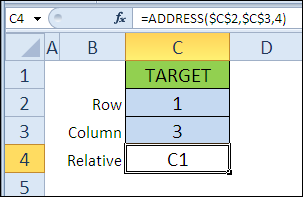##### A1 or R1C

To see R1C1 style, instead of the default A1 style, you can add FALSE in the a1 argument.

=ADDRESS(\$C\$2,\$C\$3,1,FALSE)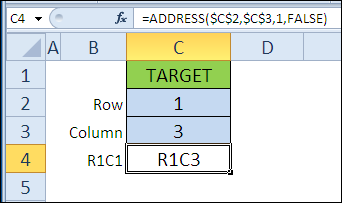##### Sheet Nam

The final argument is sheet, and you can include a sheet name, if you want it in the result.

=ADDRESS(\$C\$2,\$C\$3,1,TRUE,"Ex02")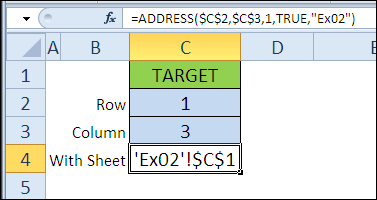### Example 2: Find cell value from row and column number

The ADDRESS function returns the cell address as text, not as an actual reference. If you want to return the cell's value, you can wrap the ADDRESS function with an INDIRECT function. We'll learn more about INDIRECT, later in the 30XL30D challenge.

=INDIRECT(ADDRESS(C2,C3))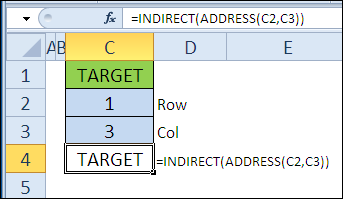The INDIRECT function will work without the ADDRESS function too. Here, the & operator is used to create an R1C1 style address, and the cell value is returned.

=INDIRECT("R" & C2 & "C" & C3,FALSE)The INDEX function can also return a cell's value, based on a row and column number.

=INDEX(1:500,C2,C3)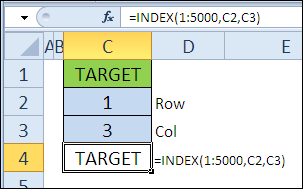### Example 3: Return address of cell with highest value

In this example, we'll find the cell with the highest value, and use the ADDRESS function to get its address.

The MAX function finds the highest number in column C.

=MAX(C3:C8)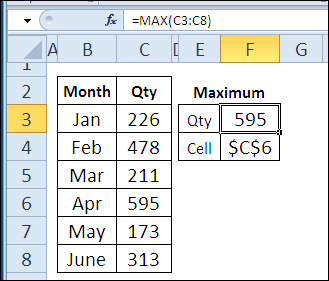Then, the ADDRESS function is combined with MATCH, which finds the row number, and COLUMN, which gets the column number.

=ADDRESS(MATCH(F3,C:C,0),COLUMN(C2))### Download the ADDRESS Function File

To see the formulas used in today's examples, you can download the ADDRESS function sample workbook. The file is zipped, and is in Excel 2007 file format.

### Watch the ADDRESS Video

To see a demonstration of the examples in the ADDRESS function sample workbook, watch this short video.

_____________

## 0 thoughts on “30 Excel Functions in 30 Days: 20 – ADDRESS”

1. dan l says:

It can be used for dynamic ranges too:

\$A\$1:(INDIRECT(ADDRESS(COUNT(Sheet1!\$A:\$A),1)))

2. Luke Wisbey says:

@dan l – off topic but if you revert to INDEX you remove Volatility from your DNR and dependants:

=Sheet1!\$A\$1:INDEX(\$A:\$A,COUNT(Sheet1!\$A:\$A))

above (like original) still susceptible to interspersed blanks; to cater for that:

=Sheet1!\$A\$1:INDEX(\$A:\$A,MATCH(9.99E+307,Sheet1!\$A:\$A))

I confess I find ADDRESS of very limited use – perhaps only where the desired result (final output) is an address string – as Debra has illustrated.

If the intention is to use the resulting Address string as the basis for another calculation there are invariably better methods.
This is perhaps more apparent where the target object is fixed given ADDRESS necessitates Volatile wrappers which could otherwise be avoided (INDEX etc).

Often people use CHAR to retrieve column letters and in so doing add lots of complexity to circumvent restriction of 26 letters whereas ADDRESS is far simpler:

=SUBSTITUTE(ADDRESS(1,A1,4),"1?,"")

where A1 holds column number

3. dan l says:

Hmmmm. Is offset a volatile too?

4. Luke Wisbey says:

Yes OFFSET is Volatile.
If interested see Charles Williams' site: http://www.decisionmodels.com/calcsecretsi.htm (and download volatilefuncs.zip)

The impact of a Volatile named range obviously depends largely on where the Named Range is to be used:

– if sourcing a Pivot or being used solely as source for Data Validation then it's of no real concern.

– if a precedent of lots of cell calculations then that would be a little different... the impact then would depend on:

a) efficiency of dependent calculation(s)

b) volume of dependent calculation(s)

c) amount of volatile actions taking place within the model

and of course

d) calculation mode

It's worth bearing in mind that OFFSET is a very fast function and INDEX is generally regards as being a little slow in comparison.
The effect of the Volatility is that though slower the dependants of the INDEX will only calculate when necessary.

In reality it boils down to implicit & explicit precedents.

Where there are implicit precedents (eg INDIRECT,OFFSET) there is invariably going to be Volatility

5. Fred Chidester says:

A little tricky – I also see here I am in some high company – I noted one thing that you need to refer, as in example 3, the whole column. Hence "C:C" because if you use this function at another point on the worksheet, the answer is incorrect. I used your example starting in "B10? with the same lay out using the "C:C" -the answer came out correct \$C\$14, however when I used both a "range name" and then tried "I11:I16? the result was "\$I\$4?. Yes it is in the fourth cell from the top of the example but, in the 14th cell in the column. Thus what happens if one would want to find the same result, as in my second example? I also used the "GoTo" to find the answer when I created my two cells – MAX and Cell at another location on the sheet. I love it it makes you think.

6. Luke Wisbey says:

@Fred, if I understand...

If we assume the I11:I16 range

=ADDRESS(MATCH(MAX(\$I\$11:\$I\$16),\$I\$11:\$I\$16,0)+ROW(\$I\$11)-1,COLUMN(\$I\$11))

If we assume a Named Range (eg _MyRange) then:

=ADDRESS(MATCH(MAX(_MyRange),_MyRange,0)+ROW(_MyRange)-1,COLUMN(_MyRange))

Generally speaking this approach is no bad thing as we are restricting the range which will minimise the work of the exact MATCH operation.

(technically the ROW & COLUMN references above will return Arrays {11;12;13;14;15;16} but only the first item will be used in this instance)

7. Contextures Blog » 30 Excel Functions in 30 Days: 28 - HYPERLINK says:

[...] different ways to create the text string for the link_location argument. In the first example, the ADDRESS function returns the address for row 1, column 1, on the sheet that is named in cell [...]

8. I'm looking up an existing cell's address in another table, so I need to return the current cell. Is it better to use =VLOOKUP(ADDRESS(A1)... or VLOOKUP(CELL("address")...? Is one better than the other?

9. Luke Wisbey says:

ADDRESS(A1) is not a valid call

To obtain the literal address of the current cell (assumed A1 – so return \$A\$1) you would use:

=ADDRESS(ROW(),COLUMN())

else

=CELL("address",A1)

the latter is Volatile whereas the former is not so I would suggest using the former
(Address also offers greater flexibility in terms of how you return the address in terms of reference style [and parent sheet object] – see XL help for more info. on the optional arguments of the ADDRESS function)

10. Thanks Luke. Sorry for the confusion – I was actually using address(row(),column()), I was just lazy in writing out my question (I should know better).

I ended up getting some strange behavior with CELL(), though I don't remember what it was, so I went with ADDRESS(). Plus, I'm trying to be better about volatility, so making that switch is a good decision anyway.

11. It can be used for efficiency of dependent calculation, volume of dependent calculation, amount of volatile actions taking place within the model and calculation

This site uses Akismet to reduce spam. Learn how your comment data is processed.